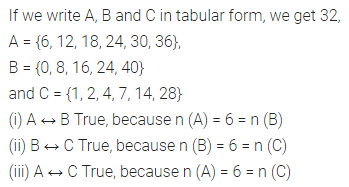# ML Aggarwal Class 7 Solutions for ICSE Maths Chapter 5 Sets Check Your Progress

## ML Aggarwal Class 7 Solutions for ICSE Maths Chapter 5 Sets Check Your Progress

Question 1.
Write the following sets in tabular form and also in set-builder form:
(i) The set of even integers which lie between -6 and 10.
(ii) The set of two-digit numbers which are a perfect square.
(iii) {factors of 42}
Solution: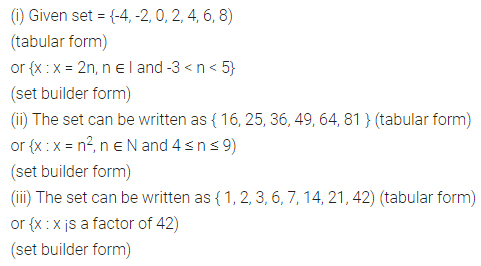Question 2.
Write the following sets in roster form:
(i) {x : x = 5n, n ∈ I and -3 < n ≤ 13}
(ii) {x : x = n2, n ∈ W and n < 5}
(iii) {x : x = n2 – 2, n ∈ W and n < 4}
Solution: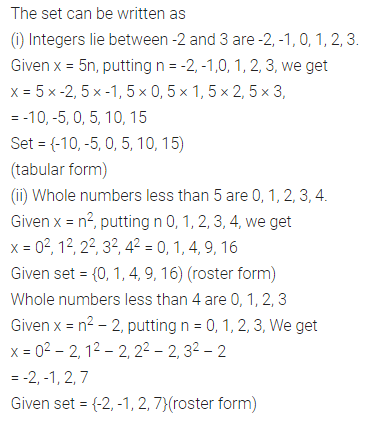Question 3.
Write the following sets in set builder form:
(i) {-14, -7, 0, 7, 14, 21, 28}
(ii) {1, 2, 3, 6, 9, 18}
Solution:Question 4.
Classify the following sets into the finite set, infinite set the empty set. In the case of a (non-empty) finite set, mention the cardinal number.
(i) The set of even prime numbers.
(ii) {multiples of 9}
(iii) {x : x is a prime factor of 84}
(iv) {x : 2x + 5 = 1, x ∈ N}
(v) {x : x is a month of a year having less than 30 days}
(vi) {x | x is a month of a leap year having 28 days}
Solution: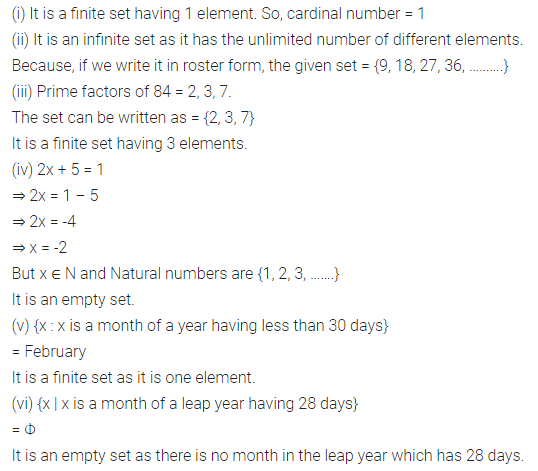Question 5.
In the following, determine whether A and B are equivalent sets and if so, whether A = B.
(i) A = {1, 3, 5}, B = {Red, Blue, Green}
(ii) A = {prime factors of 70}, B = {prime factors of 60}
(iii) A = {even natural numbers less than 10}, B = {odd natural numbers less than 10}
Solution: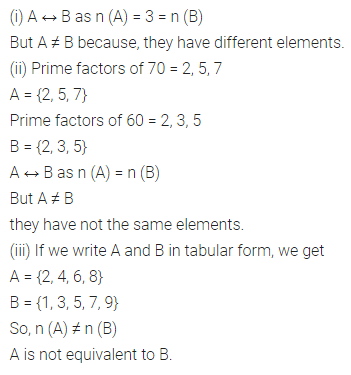Question 6.
Let P = {letters of SCHOOL} and Q = {letters of FALSE}, then State whether each of the following statement is true or false for the above sets:
(i) P ⊂ Q
(ii) Q ⊂ P
(iii) P ↔ Q
Solution:Question 7.
State whether each of the following statement is true or false for the sets A, B and C where
A = {x | x ∈ N, x < 40 and x is a multiple of 6}
B = {x | x ∈ W, x ≤ 40 and x is a multiple of 8}
C = {x | x is a factor of 28}.
(i) A ↔ B
(ii) B ↔ C
(iii) A ↔ C
Solution: